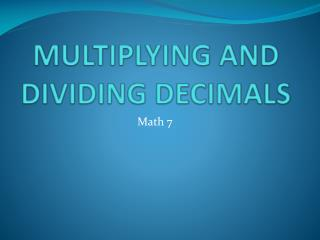Download PresentationMULTIPLYING AND DIVIDING DECIMALS

# MULTIPLYING AND DIVIDING DECIMALS - PowerPoint PPT PresentationDownload Presentation## MULTIPLYING AND DIVIDING DECIMALS

- - - - - - - - - - - - - - - - - - - - - - - - - - - E N D - - - - - - - - - - - - - - - - - - - - - - - - - - -
##### Presentation Transcript

1. MULTIPLYING DECIMALS • Multiplying Decimals to get a precise answer is a relatively simple task…assuming you know how to multiply • You don’t have to line up the decimal points like in addition and subtraction

2. MULTIPLYING DECIMALS • Multiply the numbers as if no decimal points existed • When the product is reached, it is time to place the decimal point in your answer • Count up the number of places after the decimal in each of the numbers • From the end of the product, move in that many places to the left and put the point in • Eliminate any “unnecessary zeros” on the end of the answer

3. EXAMPLES OF MULTIPLYING DECIMALS 7.29 · 2.1 14.319 · 3.18 11.2 · 0.7 Does this answer make sense? Why?

4. WORD PROBLEMS • If your speed is 3.5 miles per hour, how far will you bike in 1.2 hours? • A cubic foot of water weighs 62.4 lbs. A water storage tank can hold 89.5 cubic feet of water. How much will the water in tank weigh when the tank is full?

5. MORE WORD PROBLEMS • You have 17 pennies, 31 nickels, 22 dimes, and 14 quarters. How much money will you have left if you lend your friend \$6.50?

6. PROPERTIES OF MULTIPLICATION • Identity Property of Multiplication 6 · 1 = 6 OR x · 1 = x -The Identity Property of Multiplication states that any number times 1 will always get you the number back in return -Thus, the number 1 is called the Identity Element of multiplication

7. PROPERTIES OF MULTIPLICATION • Zero Property of Multiplication 6 · 0 = 0 OR x · 0 = 0 -The Zero Property of Multiplication states that any number times zero (0) is always going to equal zero (0) • Commutative Property of Multiplication 6 · 3 = 3 · 6 OR xy = yx -The Commutative Property of Multiplication states that when you multiply two numbers together, the order they are listed does not matter

8. PROPERTIES OF MULTIPLICATION • Associative Property of Multiplication 6 · (3 · 2) = (6 · 3) · 2 OR a(bc) = (ab)c -The Associative Property of Multiplication states that if you have three or more numbers being multiplied together, you can multiply any two at a time, and then multiply the result by the remaining number(s)

9. DIVIDING DECIMALS • Dividing decimals is also relatively easy, so long as you can perform long division. However, steps must be taken to simplify the process at the beginning of the problem. • The divisor (denominator, second number, bottom number, or the number on the “outside of the house”) must be adjusted so that no decimals are present.

10. DIVIDING DECIMALS • In order to get the divisor to be decimal free, simply move the decimal point to the right end of the number, counting how many places it moved. • Since you adjusted the divisor, the dividend (numerator, first number, number on top, or the number “inside the house”) has to be adjusted the same way. • Thus, move the decimal point just as many times as you did in the divisor. • If a decimal point still exists in the dividend, bring it up on top of the “house” directly where it is in the “house”. Divide, keeping your place values according to the point.

11. EXAMPLES OF DIVIDING DECIMALS • 4.32 ÷ 1.2 Write it in “house form” • 16.5 ÷ 1.5

12. MORE EXAMPLES OF DIVIDING DECIMALS 2.064 ÷ 0.24 144.06 ÷ 9.8

13. WORD PROBLEMS • You use 0.6 lb of bananas in each smoothie. How many smoothies can you make with 3.12 lb of bananas? • A car travels 360.25 mi. It uses 13.1 gal of gas. How many miles per gallon of gas does the car travel?

14. WORD PROBLEMS • You spend \$13.92 on fabric. Each yard costs \$4.35. How many yards of fabric did you buy? • You buy 5 movie tickets for a total of \$23.75. Your friend gives you \$5 for one ticket. How much change should you give your friend?